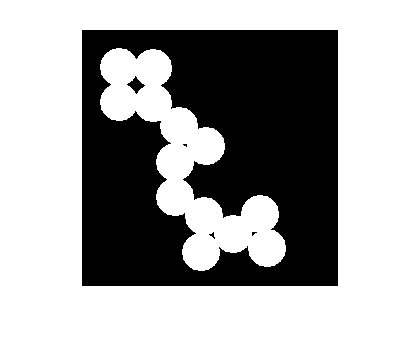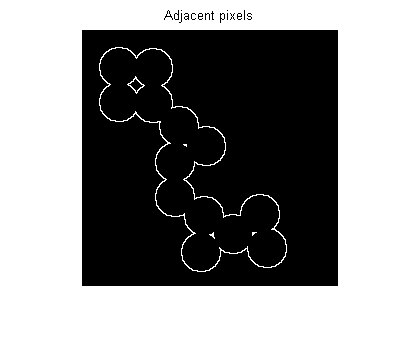## Steve on Image Processing with MATLABImage processing concepts, algorithms, and MATLAB

Here's a quick tip. A user question came in recently that involved a step of finding the pixels adjacent to foreground pixels in a binary image. Suppose you have a binary mask image, like this one:

bw = imread('circles.png');
imshow(bw)How can you find all the black pixels in bw that are immediately adjacent to a white pixel? You can do this using imdilate and a logical operation.

Use imdilate to "grow" the mask by one pixel:

bw2 = imdilate(bw, ones(3,3));

Now use a logical operation to find which pixels are white in bw2 but black in bw:

bw3 = bw2 & ~bw;
imshow(bw3)
title('Adjacent pixels')Or do it in one step:

adjacent_pixels = imdilate(bw, ones(3,3)) & ~bw;

Published with MATLAB® 7.4

|